# 0x01 背景

• Chris Eagle的The IDA Pro Book
• Alex Hanel的The Beginner’s Guide to IDAPython
• Magic Lantern 的IDAPython Wiki

# 0x02 利用IDAPython解决字符串加密问题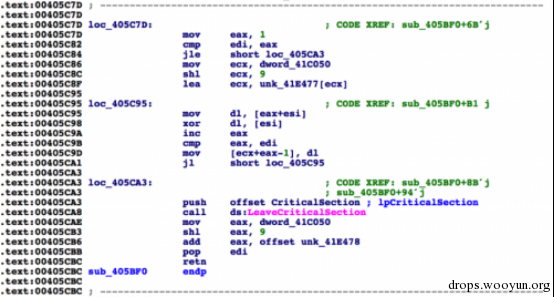图片1 字符串解密函数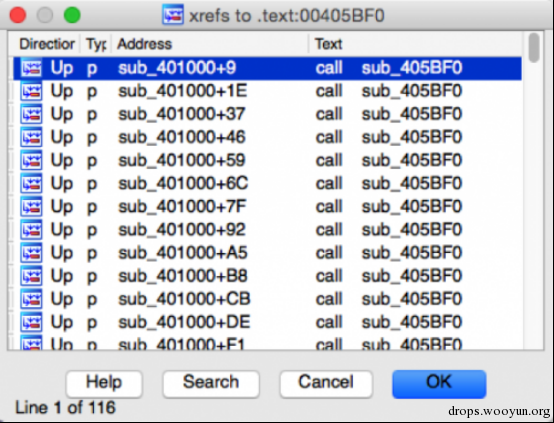图2 大量的对可疑函数的引用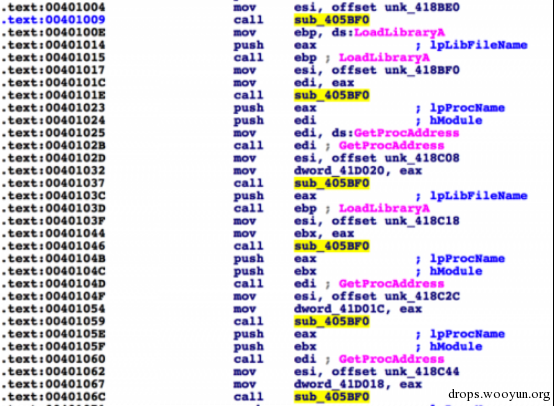图3可疑的函数 (405BF0) 被调用的实例

1. 我可以手动解密然后重命名这些字符串。
2. 我可以动态调试这个样本然后重命名遇到的字符串
3. 我可以写一个脚本用来解密并且重命名这些字符串

E4 91 96 88 89 8B 8A CA 80 88 88

``````#!python
def decrypt(data):
length = len(data)
c = 1
o = ""
while c < length:
o += chr(ord(data) ^ ord(data[c][/c]))
c += 1
return o
``````

``````#!bash
>>> from binascii import *
>>> d = unhexlify("E4 91 96 88 89 8B 8A CA 80 88 88".replace(" ",''))
>>> decrypt(d)
'urlmon.dll'
``````

``````#!python

Result:
0x401009L
0x40101eL
0x401037L
0x401046L
0x401059L
0x40106cL
0x40107fL
<truncated>
``````

``````#!python
while True:
print “We found it at 0x%x” % GetOperandValue(addr, 1)
break

Example Results:
Python>find_function_arg(0x00401009)
We found it at 0x418be0
``````

``````#!python
out = ""
while True:
else:
break
return out
``````

``````#!python
while True:
return ""

out = ""
while True:
else:
break
return out

def decrypt(data):
length = len(data)
c = 1
o = ""
while c < length:
o += chr(ord(data) ^ ord(data[c][/c]))
c += 1
return o

print "[*] Attempting to decrypt strings in malware"
for x in XrefsTo(0x00405BF0, flags=0):
ref = find_function_arg(x.frm)
string = get_string(ref)
dec = decrypt(string)
print "Ref Addr: 0x%x | Decrypted: %s" % (x.frm, dec)

Results:
[*] Attempting to decrypt strings in malware
Ref Addr: 0x401009 | Decrypted: urlmon.dll
Ref Addr: 0x401037 | Decrypted: wininet.dll
Ref Addr: 0x401046 | Decrypted: InternetOpenA
Ref Addr: 0x401059 | Decrypted: InternetOpenUrlA
<truncated>
``````

``````#!python
MakeComm(x.frm, dec)
MakeComm(ref, dec)
``````图4 运行完脚本后的字符串交叉引用界面图5 运行完脚本后的反汇编代码

# 0x03 利用IDAPython解决函数/库调用的哈希混淆问题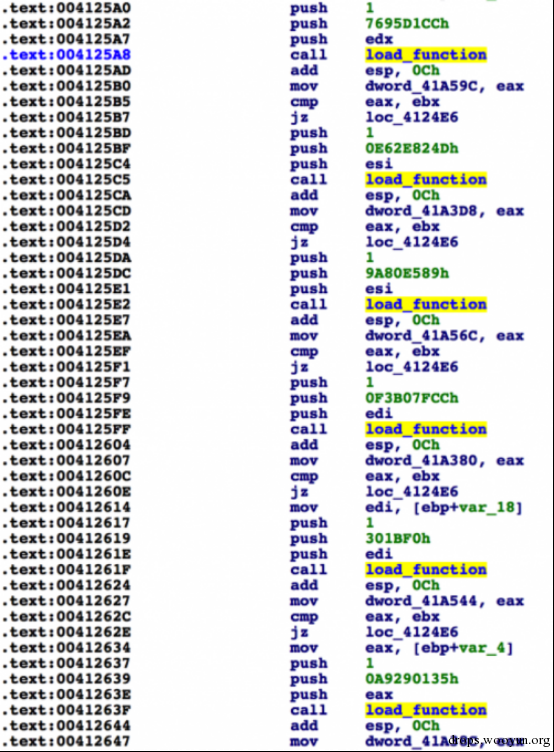图片6 malware使用CRC32哈希算法来动态的加载函数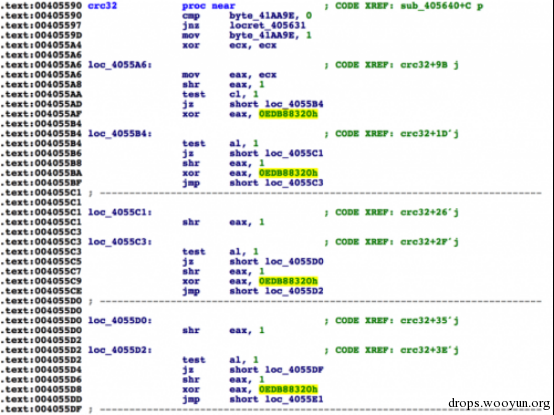图片7 确认CRC32算法

``````#!python
def get_functions(dll_path):
pe = pefile.PE(dll_path)
if ((not hasattr(pe, 'DIRECTORY_ENTRY_EXPORT')) or (pe.DIRECTORY_ENTRY_EXPORT is None)):
print "[*] No exports for %s" % dll_path
return []
else:
expname = []
for exp in pe.DIRECTORY_ENTRY_EXPORT.symbols:
if exp.name:
expname.append(exp.name)
return expname
``````

``````#!python
def calc_crc32(string):
return int(binascii.crc32(string) & 0xFFFFFFFF)
``````

``````#!bash
HASH => NAME
``````

https://github.com/pan-unit42/public_tools/blob/master/ida_scripts/gen_function_json.py

``````#!python
for k,v in json_data.iteritems():
json_data[int(k)] = json_data.pop(k)
``````

http://www.cprogramming.com/tutorial/enum.html

``````#!python
enumeration = GetEnum("crc32_functions")
if enumeration == 0xFFFFFFFF:
``````图片8 传递给load_function()的参数

``````#!python
push_count = 0
push_count += 1
if push_count == 2:
if data in json_data:
name = json_data[data]
``````

``````#!python
``````

``````#!python
def get_enum(constant):
all_enums = GetEnumQty()
for i in range(0, all_enums):
enum_id = GetnEnum(i)
enum_constant = GetFirstConst(enum_id, -1)
name = GetConstName(GetConstEx(enum_id, enum_constant, 0, -1))
if int(enum_constant) == constant: return [name, enum_id]
while True:
enum_constant = GetNextConst(enum_id, enum_constant, -1)
name = GetConstName(GetConstEx(enum_id, enum_constant, 0, -1))
if enum_constant == 0xFFFFFFFF:
break
if int(enum_constant) == constant: return [name, enum_id]
return None

enum_data = get_enum(constant)
if enum_data:
name, enum_id = enum_data
return True
else:
return False
``````图片9 当加载完函数后，程序将函数地址存储到了DWORD地址

``````#!python
MakeName(operand_value, str("d_"+name))
``````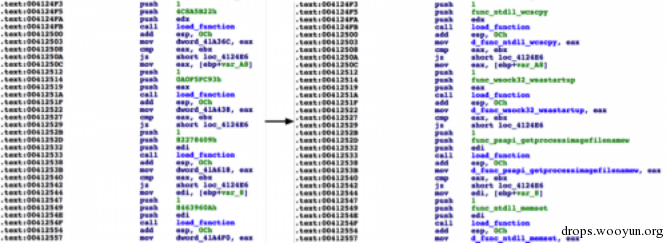图片10 运行完脚本后的变化``````#!python
import json

def get_enum(constant):
all_enums = GetEnumQty()
for i in range(0, all_enums):
enum_id = GetnEnum(i)
enum_constant = GetFirstConst(enum_id, -1)
name = GetConstName(GetConstEx(enum_id, enum_constant, 0, -1))
if int(enum_constant) == constant: return [name, enum_id]
while True:
enum_constant = GetNextConst(enum_id, enum_constant, -1)
name = GetConstName(GetConstEx(enum_id, enum_constant, 0, -1))
if enum_constant == 0xFFFFFFFF:
break
if int(enum_constant) == constant: return [name, enum_id]
return None

enum_data = get_enum(constant)
if enum_data:
name, enum_id = enum_data
return True
else:
return False

push_count = 0
push_count += 1
if push_count == 2:
if data in json_data:
name = json_data[data]
print "[+] Converted 0x%x to %s enumeration" % (current_address, name)
MakeName(operand_value, str("d_"+name))

fh = open("output.json", 'rb')
fh.close()

# JSON objects don't allow using integers as dict keys. Little workaround for
# this issue.
for k,v in json_data.iteritems():
json_data[int(k)] = json_data.pop(k)

conversion_function = 0x00405680
enumeration = GetEnum("crc32_functions")
if enumeration == 0xFFFFFFFF: